Courses

# Partial Differential Equation MCQ - 1

## 15 Questions MCQ Test Topic-wise Tests & Solved Examples for IIT JAM Mathematics | Partial Differential Equation MCQ - 1

Description
This mock test of Partial Differential Equation MCQ - 1 for Mathematics helps you for every Mathematics entrance exam. This contains 15 Multiple Choice Questions for Mathematics Partial Differential Equation MCQ - 1 (mcq) to study with solutions a complete question bank. The solved questions answers in this Partial Differential Equation MCQ - 1 quiz give you a good mix of easy questions and tough questions. Mathematics students definitely take this Partial Differential Equation MCQ - 1 exercise for a better result in the exam. You can find other Partial Differential Equation MCQ - 1 extra questions, long questions & short questions for Mathematics on EduRev as well by searching above.
QUESTION: 1

Solution:
QUESTION: 2

Solution:
QUESTION: 3

### The equation (2x + 3y)p + 4xq — 8pq = x + y is

Solution:

QUESTION: 4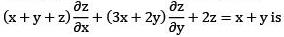Solution:
QUESTION: 5

A bounded solution to P. D. E.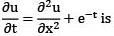Solution:
QUESTION: 6

The equation Pp + Qq + R is known as

Solution:
QUESTION: 7

The general solution of (y — z)p + (z — x)q = x — y is

Solution:
QUESTION: 8

The integral surface to the first order p. d. e 2 y (z — 3)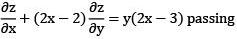through the curve x2 + y2 = 2x, z = 0 is

Solution:

QUESTION: 9

Complete integral for the partial differential equation z = px + gy — sin(pq) is

Solution:
QUESTION: 10

The general integral of the partial differential equation (y + z x) zx — (x + yz)Zy = x2 — y2 is

Solution:
QUESTION: 11

The complete integral of q(p — cosx) = cosy

Solution:
QUESTION: 12

Consider the following statement about the following p. d. e. p + q — pq = 0
(i) it has no singular solutions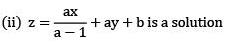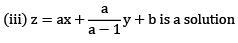(iv) It is a first order non — linear p. d. e. Then choose the
correct code.

Solution:
QUESTION: 13

Integral surface of (2x y — l)p + (z — 2x2)q = 2(x — yz) passing through the line x0 (s) = 1 y0(s) = 0 z0(s) = s

Solution:
QUESTION: 14

Integral surface of PDE x2p + y2q = —z2 which passes through hyperbola xy = x + y, z = 1 is given by

Solution:
QUESTION: 15

Consider 4xyz = pq + 2px2y + 2qxy2
(i) It can be reduced to Claerut's form by some suitable transformation.
(ii) z = ax + by + ab is complete integral
(iii) z = —x2y2 is singular solution choose correct code.

Solution: